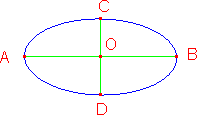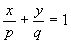www.batmath.it

A Glossary of Mathematical Terms - E

Edge

The line segment where two faces of a polyhedron meet.

EllipseOne of the conic sections.
Major (longer) axis: AB;
minor (shorter) axis: CD;
vertices: A, B, C, D;
center: O:

Empty set (Null set)

The set with nothing in it.

Endpoint

A point at the end of a line segment, a vector, a ray, ... .

Enneagon (Nonagon)

A nine-side polygon.

Equation of a straight line in a cartesian coordinate system

General form: ax + by + c = 0.
Intercept form:(x-intercept: (p,0); y-intercept: (0,q)).
Slope-Intercept form: y = mx + q (slope: m; y-intercept: (0,b)).
Point-Slope form: y - y1 = m(x - x1).
Two-Points form: (x2 - x1)(y - y1) = (y2 - y1)(x - x1)

Equiangular or Equilateral triangle

A triangle with all sides (and angles) congruent.

Even number

An integer number that is divisible by 2 without remainder.

Event

One or more outcomes of a probability experiment.

Exclusive "or"

One or the other, but not both.

Expanded numeral

A number written in a form which shows the value of the digits: 237 = (2x102) + (3x101) + (7X100)

Exponent (also called power)

In the expression ab (a to the power of b), b is the exponent.

Exponential function

A function of the form f(x) = ax.

Exterior angle

Used in polygons.

Extremes

In the proportion a:b = c:d, a and d. See Means.

first published on september 01 2003 - last updated on september 01 2003